# RRreg manual

#### 2021-09-02

Correlation and regression analysis for randomized response designs

Contact: dheck@uni-marburg.de

# Introduction

The randomized response (RR) design was developed by Warner (1965) to allow for complete anonymity of the respondents in surveys and thus to reduce effects of social desirability. In general, some kind of random noise (e.g., throwing a pair of dices) is added to the true response. Whereas the prevalence of a senstive attribute (by convention termed $$\pi$$) can still be estimated at the group level, an individual response does not disclose the true state of the respondent. Accordingly, RR data require adapted models and formulas for multivariate analysis, implemented in the package RRreg.

The main functions provide the following functionality and are explained in more detail in Section 2:

• RRuni: simple univariate analysis, i.e., prevalence estimates
• RRcor: bivariate correlations including RR variables
• RRlog: logistic regression with an RR variable as criterion
• RRmixed: logistic RR regression including random effects (nested or hierarchical data)
• RRlin: linear regression with a continuous, non-RR criterion including RR variables as predictors

Additionally, three functions can be used to generate data for robustness studies, power analysis, bootstrap estimates, and other testing purposes:

• RRgen: generates a single data frame including true states, RR responses, and group memberships
• RRsimu: Monte Carlo simulation to test RRuni,RRcor, RRlog, and RRlin either for one RR and one continuous non-RR variable or for two RR variables (only RRcor)
• powerplot: Monte Carlo simulation to plot the power of the methods RRuni,RRcor, RRlog, or RRlin either for one RR and one continuous non-RR variable

In the following, cocaine abuse is used as a running example to demonstrate the RR analysis with RRreg. The following RR designs are included in the package: UQTknown, Mangat, Kuk, FR, Crosswise, Triangular, UQTunknown, CDM, CDMsym, SLD

# Statistical Methods

### Univariate Analysis

The original RR design proposed by Warner in 1965 works as follows: With randomization probability p, participants are supposed to answer to the question ‘Have you ever used cocaine?’, and with counter-probability 1-p, the question is reversed, i.e., ‘Have you never used cocaine?’. As a randomization device, dices or coin flips with known probabilities may be used. The model is depicted in the following figure: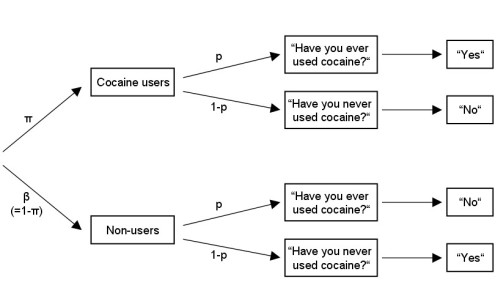First, let’s load the package and generate a single data frame using the function RRgen. We simulate data for 1000 participants, a proportion of 30% cocaine users (pi.true=.3; obviously, this rate is unrealistic, but it should suffice for the sake of example), and a randomization probability of p=.2:

library(RRreg)
data.W <- RRgen(n=1000, pi.true=.3, model="Warner", p=.2,
complyRates = c(1, 1), sysBias = c(0,0))
head(data.W)
##   true comply response
## 1    0      1        0
## 2    0      1        1
## 3    0      1        1
## 4    0      1        1
## 5    1      1        1
## 6    0      1        0

In the function RRgen, the argument complyRates gives the proportions of participants who do adhere to the RR instructions for carriers and non-carriers of the sensitive attribute, respectively. The argument sysBias gives the probability of yes responses in case of non-compliance for carriers and non-carriers, respectively. If sysBias=c(0,0), noncomplying individuals always respond with self-protective no responses (also known as SP-‘no’ responses). In comparison, sysBias=c(0,0.5) indicates that noncomplying non-carriers answer yes or no by chance. However, since we generated data assuming perfect compliance, this argument can be neglected. These options can be used to test how robust the results are against non-adherence.

Now that we have a data set, we can estimate the prevalence of cocaine users by means of RRuni:

warner <- RRuni(response=response, data=data.W,
model="Warner", p=.2, MLest=T)
summary(warner)
## Warner Model with p = 0.2
## Sample size: 1000
##
##    Estimate   StdErr      z  Pr(>|z|)
## pi 0.268333 0.025326 10.595 < 2.2e-16 ***
## ---
## Signif. codes:  0 '***' 0.001 '**' 0.01 '*' 0.05 '.' 0.1 ' ' 1

The response variable containing 1 for yes and 0 for no responses can either be given as a vector or as a column name in the data set data. Moreover, the argument model specifies the RR design with its randomization probability given by p (see Sections 4 and 5 for details about other RR models) . If MLest=FALSE is used, the prevalence estimate is derived as an analytical least-squares estimator, which can assume values outside of the interval [0,1]. However, using MLest=TRUE (default), estimates of pi are restricted to the interval [0,1].

• Warner, S. L. (1965). Randomized response: A survey technique for eliminating evasive answer bias. Journal of the American Statistical Association, 60, 63-69.

### Multivariate Analysis

First, we generate a continuous, non-RR variable. According to our simulation, respondents carrying the sensitive attribute (i.e., cocaine users) have higher scores on this covariate:

data.W$cov[data.W$true==1] <- rnorm(sum(data.W$true == 1),1) data.W$cov[data.W$true==0] <- rnorm(sum(data.W$true == 0))

#### RRcor: RR correlations

Now, we can estimate the bivariate (point-biserial) correlation between the dichotomous Warner RR variable and the continuous covariate:

RRcor(x=data.W$response, y=data.W$cov, models=c("Warner", "direct"),
p.list=list(.2), bs.n=0, bs.type = c("se.n", "se.p", "pval"), nCPU=1)
## Randomized response variables:
##   Variable        RRmodel p
## 1 data.W$response Warner 0.2 ## 2 data.W$cov      direct
##
## Sample size N = 1000
##
## Estimated correlation matrix:
##                 data.W$response data.W$cov
## data.W$response 1.000000 0.416216 ## data.W$cov             0.416216   1.000000

The argument models defines which variables given by x and y are treated as RR variables. x and y can be vectors and/or data frames/matrices having one variable in each column (the order is then given as following: First, columns of x from left to right, and second, columns of y from left to right). The randomization probabilities p.list have to be in the same order as models. No values have to be specified for direct (nonRR) variables. To obtain bootstrapped standard errors, bs.n has to be set to a number of bootstrap replications larger than 0. Additionally, bs.type defines which kind of bootstrap should be performed ("se.n", "se.p": nonparametric/parametric for standard errors; "pval" parametric bootstrap assuming independence for p-values). For faster processing, nCPUcan be increased to use parallel computation. In our example, we also know the true states of the participants in the data set and can check the results by:

cor(x=data.W$true, y=data.W$cov)
##  0.4167635
• Fox, J. A., & Tracy, P. E. (1984). Measuring associations with randomized response. Social Science Research, 13, 188-197.

#### RRlog: Logistic RR Regression

Alternatively, we can run a logistic regression to predict the probability of cocaine use. In the formula below, the Warner RR variable is defined as criterion on the left side, whereas the continuous covariate is used as predictor on the right. The interface is similar to that of the standard linear regression in R (i.e., lm()).

log1 <- RRlog(formula=response~cov, data=data.W,
model="Warner", p=.2, LR.test=TRUE, fit.n = 1)
summary(log1)
## Call:
## RRlog.formula(formula = response ~ cov, data = data.W, model = "Warner",
##     p = 0.2, LR.test = TRUE, fit.n = 1)
##
## RR Model:
## Warner with p =  0.2
##
## Model fit:
##     n    logLik
##  1000 -625.7062
##
##             Estimate   StdErr Wald test Pr(>Chi2,df=1) deltaG2 Pr(>deltaG2)
## (Intercept) -1.50593  0.21409  49.47638        0.00000  110.27    < 2.2e-16 ***
## cov          1.04870  0.18593  31.81188        0.00000   56.57    < 2.2e-16 ***
## ---
## Signif. codes:  0 '***' 0.001 '**' 0.01 '*' 0.05 '.' 0.1 ' ' 1

To remove the intercept from the model, use formula=response~cov - 1. The argument LR.test determines whether the coefficients should be tested by means of a likelihood ratio test, that is, by removing one predictor at a time. Again, we can check the results by:

glm(formula=true~cov, data=data.W, family=binomial(link = "logit"))
##
## Call:  glm(formula = true ~ cov, family = binomial(link = "logit"),
##     data = data.W)
##
## Coefficients:
## (Intercept)          cov
##      -1.440        1.037
##
## Degrees of Freedom: 999 Total (i.e. Null);  998 Residual
## Null Deviance:       1180
## Residual Deviance: 990.4     AIC: 994.4
• van den Hout, A., van der Heijden, P. G., & Gilchrist, R. (2007). The logistic regression model with response variables subject to randomized response. Computational Statistics & Data Analysis, 51, 6060-6069.

#### RRmixed: Logistic RR Regression with Mixed Effects

If the data are nested (e.g., students within schools), the logistic regression can be extended to include random effects. In R, the package lme4 allows for flexible generalized linear mixed models (GLMMs) which include RR logistic regression as a special case. The function RRmixed uses the function glmerof lme4 and an adjusted link function. However, at the moment this can only be used with one-group RR models that assume the same link function for all responses.

Some typical examples how to specify models fia the formula are:

• random effects: rrt ~ covariate + (1 | cluster)
• random slopes: rrt ~ covariate + (0+covariate | cluster)
• both: rrt ~ covariate +(covariate | cluster)
• level-2 predictors: rrt ~ covariate + lev2pred + (1 | cluster) (the predictor lev2pred must have constant values within clusters)

The function RRmixed is used as follows:

# make random cluster:
data.W$cluster <- c("a", "b") mixmod <- RRmixed(response ~ cov + (1 | cluster), data=data.W, model = "Warner", p = .2) ## boundary (singular) fit: see ?isSingular mixmod ## Generalized linear mixed model fit by maximum likelihood (Laplace ## Approximation) [glmerMod] ## Family: binomial ( logRR(0.8,0.2) ) ## Formula: response ~ cov + (1 | cluster) ## Data: data.W ## AIC BIC logLik deviance df.resid ## 1257.4123 1272.1356 -625.7062 1251.4123 997 ## Random effects: ## Groups Name Std.Dev. ## cluster (Intercept) 0 ## Number of obs: 1000, groups: cluster, 2 ## Fixed Effects: ## (Intercept) cov ## -1.506 1.049 ## optimizer (Nelder_Mead) convergence code: 0 (OK) ; 0 optimizer warnings; 1 lme4 warnings • van den Hout, A., van der Heijden, P. G., & Gilchrist, R. (2007). The Logistic Regression Model with Response Variables Subject to Randomized Response. Computational Statistics & Data Analysis, 51, 6060–6069. #### RRlin: RR Predictors in a Linear Regression Third, we can use the Warner RR variable as predictor in a linear regression: lin1 <- RRlin(formula=cov~response, data=data.W, models="Warner", p.list=.2, bs.n=0, fit.n=1) summary(lin1) ## Call: ## RRlin(formula = cov ~ response, data = data.W, models = "Warner", ## p.list = 0.2, bs.n = 0, fit.n = 1) ## ## Randomized response variables: ## Variable Model p ## 1 response Warner 0.2 ## ## Coefficients (beta): ## Estimate StdErr Wald test Pr(>Chi2,df=1) ## (Intercept) -0.03420 0.04611 0.5502 0.4582 ## response 1.02334 0.12585 66.1188 <2e-16 *** ## --- ## Signif. codes: 0 '***' 0.001 '**' 0.01 '*' 0.05 '.' 0.1 ' ' 1 ## ## Residual standard error (sigma): 0.988; N=1000 As in RRcor, bootstrapped standard errors can be obtained by setting bs.n to the number of bootstrap replications. The order of the RR models is defined by models and p.list. For instance, given three RR variables rr1, rr2, and rr3 and two non-RR/direct variables x1 and x2, the model could be specified by formula = y ~ rr1 + x1 + rr2 + rr3 + x2 and models=c('Warner', 'direct', 'Warner', 'Warner', 'direct'). Note that the predictors in formula can either be vectors or column names of variables in the data frame data. As a special case, if the number of predictors used in the formula interface is larger than the number of RR variables specified in models, the remaining predictors are treated as directly measured, non-RR variables: data.W$pred <- rnorm(1000)
lin2 <- RRlin(formula=cov~response + pred, data=data.W,
models="Warner", p.list=list(.2), bs.n=0, fit.n=1)
summary(lin2)
## Call:
## RRlin(formula = cov ~ response + pred, data = data.W, models = "Warner",
##     p.list = list(0.2), bs.n = 0, fit.n = 1)
##
## Randomized response variables:
##   Variable Model  p
## 1 response Warner 0.2
##
## Coefficients (beta):
##             Estimate   StdErr Wald test Pr(>Chi2,df=1)
## (Intercept) -0.03434  0.04610    0.5547         0.4564
## response     1.02300  0.12578   66.1491         <2e-16 ***
## pred        -0.01104  0.03293    0.1124         0.7374
## ---
## Signif. codes:  0 '***' 0.001 '**' 0.01 '*' 0.05 '.' 0.1 ' ' 1
##
## Residual standard error (sigma): 0.988; N=1000

To check the results of the simulation, run:

lm(cov~true + pred, data=data.W)
##
## Call:
## lm(formula = cov ~ true + pred, data = data.W)
##
## Coefficients:
## (Intercept)         true         pred
##   -0.041161     1.012624     0.006078

It is also possible to include several RR variables as predictors if the randomization devices used are stochastically independent:

data.W2 <- RRgen(n=1000, pi.true=.45, model="Warner", p=.35)
data.W$cov <-2*data.W$true - 3*data.W2$true+ rnorm(1000,1,5) data.W$response2 <- data.W2$response lin3 <- RRlin(cov ~ response + response2, data=data.W, models=c("Warner", "Warner"), p.list=list(.2, .35), fit.n=1) summary(lin3) ## Call: ## RRlin(formula = cov ~ response + response2, data = data.W, models = c("Warner", ## "Warner"), p.list = list(0.2, 0.35), fit.n = 1) ## ## Randomized response variables: ## Variable Model p ## 1 response Warner 0.2 ## 2 response2 Warner 0.35 ## ## Coefficients (beta): ## Estimate StdErr Wald test Pr(>Chi2,df=1) ## (Intercept) 0.79347 0.55403 2.0511 0.15209 ## response 2.62414 0.92156 8.1082 0.00441 ** ## response2 -3.26164 1.19132 7.4957 0.00618 ** ## --- ## Signif. codes: 0 '***' 0.001 '**' 0.01 '*' 0.05 '.' 0.1 ' ' 1 ## ## Residual standard error (sigma): 4.904; N=1000 Besides the regression output, the function summary also provides ‘Prevalence estimates for combinations of RR responses’. In our example using two Warner variables, the combination 0:0 indicates the sub-group having neither of both sensitive attributes and shows the corresponding prevalence estimate. Similarly, the combination 0:1 indicates the sub-group having only the second sensitive attribute and so on. Note that the last combination 1:1 is not provided and can be calculated by summing up all other prevalence estimates and substracting this sum from 1. To include many RR variables as predictors, very large sample sizes are required for reliable estimation. In such a case, it might be necessary to adjust the optimization parameters maxit (maximum number of iterations) and pibeta (relative ratio of proability scale for pi to regression coefficients beta; e.g., choose a smaller value for larger absolute beta values). • van den Hout, A., & Kooiman, P. (2006). Estimating the linear regression model with categorical covariates subject to randomized response. Computational Statistics & Data Analysis, 50, 3311-3323. # Testing RR vs. Direct Questioning (DQ) The method RRlog can be used to test whether an RR design leads to higher prevalence estimates than direct questioning (DQ). For one-groups designs (such as the Warner model), define a vector with indices 0 for all participants who were questioned by DQ and indices 1 for participants questioned by the RR design and include this dummy variable as predictor. For two-group designs (see below), define an effect-coded variable contrasting DQ ($$=-1$$) and RR ($$=+1$$), and define a vector with indices 0 for participants questioned by DQ and indices 1 and 2 denoting the group of the RR design. If the regression coefficient of the effect-coded predictor is significant, this indicates different prevalence rates between RR and DQ. Furthermore, interactions between question format (RR vs. DQ) and other predictors can be tested. For instance, one might want to know whether the regression coefficient of a predictor (e.g., age) differs between question formats (e.g., whether the RR format serves as a more valid criterion). Interactions are included by formula= RR ~ format + age + format:age. An example is: # generate data with different prevalence rates for RR and DQ RR <- RRgen(n = 500, pi = .5, model = "Warner", p = 2/12) DQ <- rbinom(500, 1, prob = .35) response <- c(RR$response, DQ)
# dummy variable for RR vs. DQ
group <- rep(1:0, each=500)
# predictor variable (effect-coded)
RR.design <- rep(c(1,-1), each=500)
# simulate interaction of question format and age
# (DQ: no correlation; RR: positive correlation)
z.age <- scale(c(rnorm(500, mean=RR$true, sd=3), rnorm(500, mean=.5, sd=3))) # fit full model # (testing difference in prevalence estimates and interaction) fit <- RRlog(response ~ RR.design * z.age, model="Warner", p=2/12, group=group, LR.test=TRUE, fit.n = 1)  ## Warning: The group vector contains 2 categories, which are interpreted ## asfollows: 0=direct question format ; 1=randomized response format summary(fit) ## Call: ## RRlog.formula(formula = response ~ RR.design * z.age, model = "Warner", ## p = 2/12, group = group, LR.test = TRUE, fit.n = 1) ## ## RR Model: ## Warner with p = 0.167 (n=500) combined with DQ (n=500) ## ## Model fit: ## n logLik ## 1000 -667.8259 ## ## Estimate StdErr Wald test Pr(>Chi2,df=1) deltaG2 Pr(>deltaG2) ## (Intercept) -0.31796 0.08354 14.48741 0.00014 14.4339 0.00015 ## RR.design 0.25584 0.08354 9.37978 0.00219 9.2239 0.00239 ## z.age 0.26898 0.08656 9.65652 0.00189 10.3764 0.00128 ## RR.design:z.age 0.14589 0.08656 2.84078 0.09190 2.9673 0.08496 ## ## (Intercept) *** ## RR.design ** ## z.age ** ## RR.design:z.age . ## --- ## Signif. codes: 0 '***' 0.001 '**' 0.01 '*' 0.05 '.' 0.1 ' ' 1 # get prevalence estimate for RR and DQ # (for z.age = 0 = mean(z.age)) logit1 <- coef(fit) %*% c(1, 1, 0, 1*0) cat(exp(logit1) / (1+ exp(logit1))) ## 0.4844757 logit2 <- coef(fit) %*% c(1, -1, 0, -1*0) cat(exp(logit2) / (1+ exp(logit2))) ## 0.3603599 # Specification of RR Models Using One Group In the following, several other available RR models are listed, which can be used throughout the package functions. For each model, the randomization probability p must be given as defined below. #### Unrelated Question Techique with Known Prevalance (UQTknown) model = 'UQTknown' In this RR design, participants either respond to the sensitive question or to a second irrelevant question. Importantly, the interviewer is unaware which question was answered. The randomization probability p determines the proportion of participants that is directed to answer the sensitive question, whereas the remaining participants answer the irrelevant question. In the UQTknown design, the prevalence rate of yes responses for this irrelevant question must be known beforehand (e.g., “Is your birthday in April?”, p=1/12) and provided together with the randomization probability by the vector p=c(p, p) (see UQTunknown if the prevalence is unknown).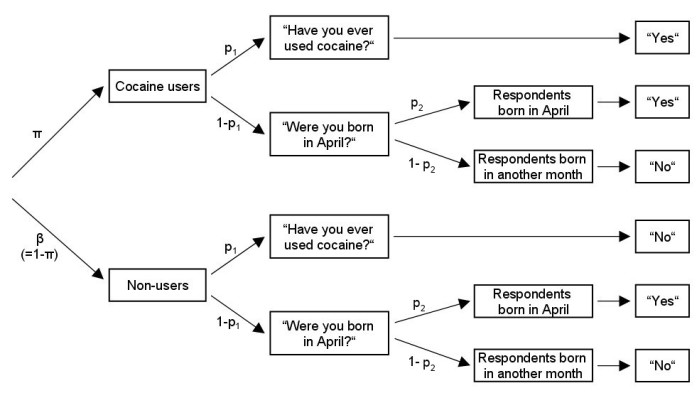• Scheers, N. J. (1992). A review of randomized response techniques. Measurement and Evaluation in Counseling and Development, 25, 27-41. #### Mangat’s RR Design model = 'Mangat' In Mangat’s model, carriers of the critical attribute are always provided with the sensitive statement. In contrast, non-carriers of the critical attribute are to respond to the sensitive statement with probability p and to its negation otherwise.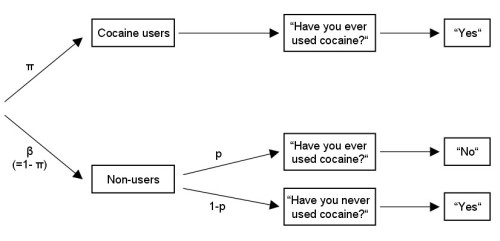• Mangat, N. (1994). An improved randomized-response strategy. Journal of the Royal Statistical Society, Series B, 56, 93-95. #### Kuk’s RR Design model = 'Kuk' Kuk’s method uses two decks of playing cards to ensure anonymity of the respondents. Whereas carriers of the sensitive attribute are told to report the color of a card drawn from the first deck, non-carriers should report the color of a card from the second deck. Importantly, the interviewer does not know, from which deck a card was drawn. Each deck contains red and black cards, coded with 1 and 0, respectively. However, the proportion of red cards differs in the two decks, defined by the vector p=c(p, p) (i.e., proportion of red cards for carriers and non-carriers of the sensitive attribute, respectively). Note that the procedure can be applied repeatedly to obtain more reliable estimates (with replacement of the drawn cards). For instance, with 5 repetitions, the observed RR variable can assume values from 0 to 5, i.e., the reported number of red cards. The functions RRgen (data generation), RRuni (univariate analysis), and RRlin (linear regression with RR predictors) can accomodate this extended design, using the additional argument Kukrep. However, at the moment, this option is not available in RRcor and RRlog.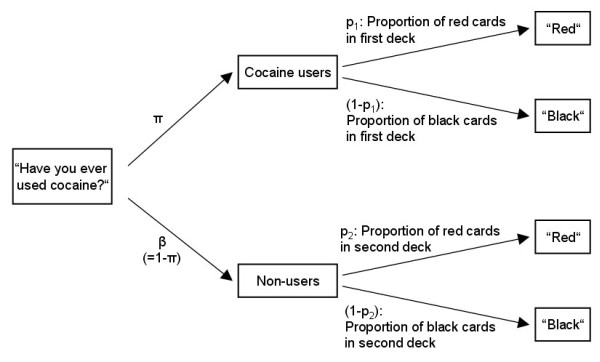• Kuk, A. Y. (1990). Asking sensitive questions indirectly. Biometrika, 77, 436-438. #### Forced Response (FR) model = 'FR' In the forced response (FR) model (also known as directed-answers model), a known proportion of the participants is prompted to respond yes, whereas another proportion is prompted to respond no, independent of their true status. Only the remaining participants answer truthfully. The randomization probability p is now a vector, containing two values, i.e., being prompted to respond no and yes, respectively. The model is shown in the following figure: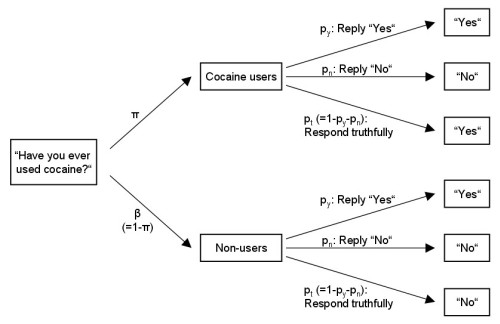The FR model can easily be extended to account for more than two response categories. For instance, responses can be given on a 5-point Likert scale from 0 to 4. For these categories, the randomization probabilities, determining the proportion of forced responses, are provided in a vector p=c(p, p,...,p). The sum of these probabilities must be smaller than one. This polytomous RR model can be used with the functions RRgen, RRuni, RRcor, and RRlin (the logistic RR regression RRlog requires a dichotomous response format). The following code snippets illustrate, how the FR method is used with multiple response categories: • RRgen(n=1000, pi.true=c(.3,.1,.4,.2), model="FR", p=c(.1,.1,.2,.05)) • RRlin(criterion~response, data=dat, models="FR",p.list=list(c(.1,.1,.2,.05)), fit.n=1) For data generation of a polytomous FR model using RRgen, the arguments complyRates and sysBias have the following definition: • complyRates=c(c0, c1, ...) gives the probability of complying to the RR instructions separately for all possible true states • sysBias = c(s1, s2, ...) defines the multinomial probability distribution which is used in case of noncompliance (the values have to sum up to 1). For instance, having the categories 0,1,2,3,4 and complyRates=c(1,1,1,.8,.5), sysBias=c(.5,.3,.2,0,0) would simulate the scenario that participants with true states 3 and 4 would comply less and instead prefer responses 0, 1, or 2. • Greenberg, B. G., Abul-Ela, A.-L. A., Simmons, W. R., & Horvitz, D. G. (1969). The Unrelated Question Randomized Response Model: Theoretical Framework. Journal of the American Statistical Association, 64, 520-539. #### Crosswise Model model = 'Crosswise' Concerning the model equations, the Crosswise model is technically identical to the Warner model. However, instead of answering to the sensitive question directly, respondents are instructed to respond to the sensitive and an irrelevant question simultaneously, according to the following scheme: • If your answer is yes to both questions or no to both questions, choose Option A (coded as 1) • If your answer is yes to one and no to the other question, choose Option B (coded as 0)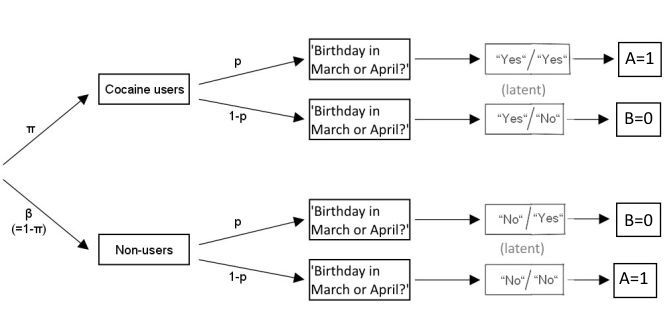For the irrelevant question, the prevalence of yes responses has to be known beforehand and is specified in RRreg by p (e.g., for the irrelevant question ‘Is Your birthday in March or April?’, p = 2/12). • Yu, J.-W., Tian, G.-L., & Tang, M.-L. (2008). Two new models for survey sampling with sensitive characteristic: Design and analysis. Metrika, 67, 251-263. #### Triangular Model model = 'Triangular' The Triangular model is technically similar to the Mangat’s RR model. However, instead of answering to the sensitive question directly, respondents are instructed to respond to the sensitive and an irrelevant question simultaneously, according to the following scheme: • If your answer is no to both questions, respond with Option A (coded as 0, the circle’ in Yu et al., 2008) • If your answer is yes to at least one of the two questions, respond with Option B (coded as 1; the triangle’ in Yu et al., 2008) For the irrelevant question, the prevalence of yes responses has to be known beforehand and is specified in RRreg by p (e.g., for the irrelevant question ‘Is Your birthday in March or April?’, p = 2/12). • Yu, J.-W., Tian, G.-L., & Tang, M.-L. (2008). Two new models for survey sampling with sensitive characteristic: Design and analysis. Metrika, 67, 251-263. #### RR as Misclassification model = 'custom' RR designs can be understood as a misclassification mechanisms: The true latent states/responses are perturbed randomly according to a known randomization scheme. This randomization scheme can be described in form of a matrix $$PW$$, where each entry $$PW_{ji}$$ gives the probability to give a response $$j$$ if the true state is $$i$$. The package RRreg contains the function getPW to obtain this misclassification matrix for the implemented designs. The forced response design with proabilities P=c(.1,.2), for instance, can be described by: getPW(model = "FR", p = c(.1, .1)) ## 0 1 ## 0 0.9 0.1 ## 1 0.1 0.9 To specify a custom misclassification matrix, the arguments model = "custom" and a randomization matrix p=matrix(p00, p10, p01, p11), ncol=2, nrow=2) are required. The approach generalizes to categorical, non-binary data by specifying an $$m \times M$$ matrix. Note that for two-group designs, the matrix $$PW$$ depends on the groupd and on a second parameter, which needs to be estimated from the data (e.g., the unknown prevalence in "UQTunknown"). • van den Hout, A., & Kooiman, P. (2006). Estimating the Linear Regression Model with Categorical Covariates Subject to Randomized Response. Computational Statistics & Data Analysis, 50(11), 3311–3323. # Specification of RR Models Using Two Groups For several reasons, RR designs were proposed that require two independent random samples (groups). Historically, the second group was used to estimate the prevalence of the irrelevant question in the UQT. Alternatively, two groups allow for a second parameter, quantifying cheating or compliance with the RR procedure. For these two-group models, the functions RRuni, RRcor, RRlog, and RRlin additionally require a vector group with values in 1 and 2, indicating the group membership of each respondent. If more than one RR variable is in the model, group is specified by a matrix containing the corresponding group vectors (one in each column) in the same order as the RR variables appear in the model. Moreover, the randomization probability p often specifies different values for the two groups. Code examples are given in Section 5.5. Note that in the functions RRcor and RRlin, the second parameter of these two-group models is estimated first and then treated as a fixed constant in further computations. For the maximum likelihood estimation in RRlin, the second parameter should ideally be included as an additional, free parameter to provide unbiased standard errors (this is the case for the function RRlog). However, simulations show that the results are still adequate. RRsimu can be used to run such a simulations yourself and get bootstrapped standard errors. #### Unrelated Question Technique with Unknown Prevalance (UQTunknown) model = 'UQTunknown' In this extension of the UQTknown RR design, the prevalence of the second, irrelevant question is unknown beforehand and estimated from the data. Note that this second question has to be stochastically independent of the sensitive attribute. The randomization probability p is defined as • p - probability to respond to the sensitive question in group 1 • p - probability to respond to the sensitive question in group 2 The randomization probabilities must differ across groups to render the model identifiable (i.e., p!=p). When generating data by means of RRgen, the prevalence rates of yes responses can be specified by pi=c(pi.sensitive, pi.irrelevant). If only a single value is provided, the prevalence rate for the irrelevant question is randomly drawn from a uniform distribution. Note that Moor’s variant of the UQT with unknown prevalence can be used by setting p=0. Thereby, the second group is only used to estimate the prevalence of responding yes to the irrelevant question.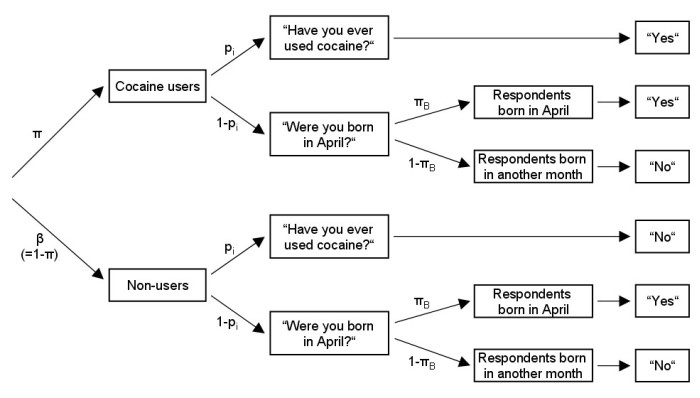• Greenberg, B. G., Abul-Ela, A.-L. A., Simmons, W. R., & Horvitz, D. G. (1969). The Unrelated Question Randomized Response Model: Theoretical Framework. Journal of the American Statistical Association, 64, 520-539. • Moors, J. J. A. (1971). Optimization of the Unrelated Question Randomized Response Model. Journal of the American Statistical Association, 66, 627-629. #### Cheating Detection Model (CDM) model = 'CDM' The CDM is based on the forced-response design and prompts a proportion p of participants to respond yes regardless of their true state. In contrast to the FR design, the cheating detection model (CDM) divides the population into three distinct groups: Compliant carriers of the sensitive attribute (pi), compliant noncarriers (beta), and cheating respondents who always respond no (gamma). This last group of cheaters is not further divided into carriers and noncarriers of the sensitive attribute. To estimate the two parameters pi and gamma, the sample is randomly divided into two parts using different randomization probabilities defined as: • p - probability to be prompted to say yes in group 1 • p - probability to be prompted to say yes in group 2 The randomization probabilities must differ across groups to render the model identifiable (i.e., p!=p). Because of the assumption of three distinct groups, the CDM is not available in the functions RRcor and RRlin. However, the function RRlog performs a logistic regression to predict the probability of being in the first group (i.e., having the sensitive attribute and complying with the RR procedure). Note that conclusions are restricted to this group, only.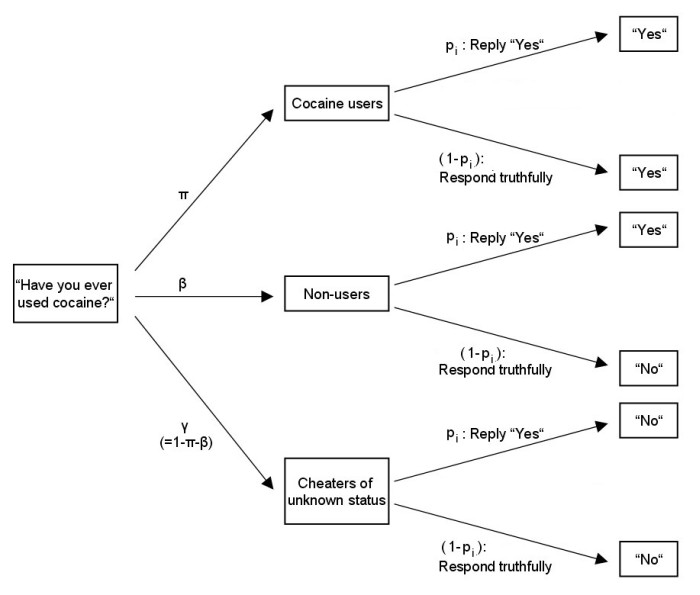• Clark, S. J., & Desharnais, R. A. (1998). Honest answers to embarrassing questions: Detecting cheating in the randomized response model. Psychological Methods, 3, 160-168. #### Symmetric CDM (CDMsym) model = 'CDMsym' In contrast to the standard cheating detection model (CDM) discussed in Section 5.2, in the symmetric CDM (CDMsym), some respondents are also prompted to respond no with a given probability, not only yes. Thereby, anonymity and compliance can be increased (Ostapczuk & al., 2009), because participants directly see that both, yes and no responses are uninformative in respect to the sensitive attribute. Similarly as with the CDM, using CDMsym in RRlog predicts the joint probability of having the sensitive attribute and complying with the RR procedure. CDMsym cannot be used in the functions RRcorand RRlin. The randomization probability p is a vector with 4 values: • p - probability to be prompted to say yes in group 1 • p - probability to be prompted to say no in group 1 • p - probability to be prompted to say yes in group 2 • p - probability to be prompted to say no in group 2 The randomization probabilities must meet these restrictions: • different probabilities across groups (p!=pand p!=p) • within each group, the sum of both probabilities is below one (p+p<1 and p+p<1)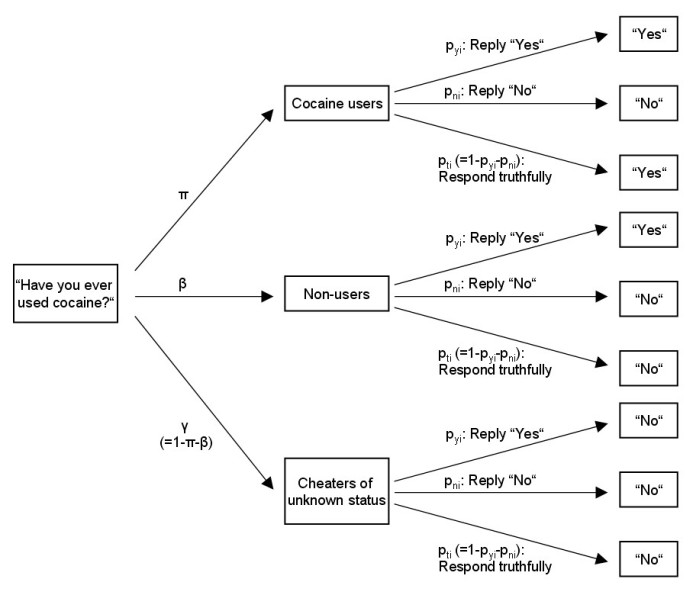• Ostapczuk, M., Moshagen, M., Zhao, Z., & Musch, J. (2009). Assessing sensitive attributes using the randomized response technique: evidence for the importance of response symmetry. Journal of Educational and Behavioral Statistics, 34, 267-287. #### Stochastic Lie Detector (SLD) model = 'SLD' The stochastic lie detector (SLD) is based on Mangat’s model (Section 4.2) and adds a second parameter t, which gives the probability of honest responding within the group carrying the sensitive attribute. Note that in contrast to the CDM, the probability of having the sensitive attribute pi is corrected for cheating. The randomization probability p is a vector with 2 values: • p - probability for noncarriers to reply with no in group 1 • p - probability for noncarriers to reply with no in group 2 The randomization probabilities must differ across groups to render the model identifiable (i.e., p!=p).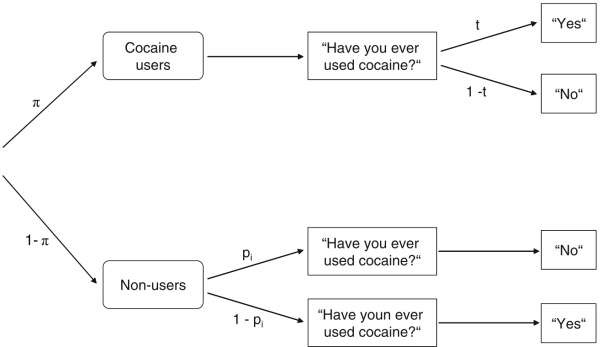• Moshagen, M., Musch, J., & Erdfelder, E. (2012). A stochastic lie detector. Behavior Research Methods, 44, 222-231. #### Code Examples for Two-Group Models ### generate 2 two-group RR variables and a continuous covariate RR1 <- RRgen(1000, pi=.4, model="SLD", p=c(.2,.8), complyRates=c(.8,1)) RR2 <- RRgen(1000, pi=.6, model="SLD", p=c(.3 ,.7), complyRates=c(.9,1)) cov <- RR1$true + RR2$true + rnorm(1000, 0, 3) ### logistic regression (dependent RR variable) logmod <- RRlog(RR1$response ~ cov, model="SLD", p=c(.2,.8),
group=RR1$group, fit.n = 1) summary(logmod) ## Call: ## RRlog.formula(formula = RR1$response ~ cov, model = "SLD", p = c(0.2,
##     0.8), group = RR1$group, fit.n = 1) ## ## RR Model: ## SLD with p = 0.2,0.8 ## ## Model fit: ## n logLik ## 1000 -577.9825 ## ## Estimate StdErr Wald test Pr(>Chi2,df=1) deltaG2 Pr(>deltaG2) ## (Intercept) -0.82045 0.23056 12.66281 0.00037 14.6272 0.00013 *** ## cov 0.07946 0.04911 2.61803 0.10566 2.6871 0.10117 ## t 0.85130 0.05797 6.57943 0.01032 4.3946 0.03605 * ## --- ## Signif. codes: 0 '***' 0.001 '**' 0.01 '*' 0.05 '.' 0.1 ' ' 1 ## ## Note that the parameter t is tested against the null hypothesis ## that all participants answered truthfully (i.e., H0: t=1). ### group matrix for RRlin and RRcor: use multiple group vectors in one matrix group <- cbind(RR1$group, RR2$group) # bivariate correlation between 2 two-group variables and a continuous covariate # note that only the most informative subsets of the data are used (see ?RRcor) responses <- cbind(RR1$response, RR2$response, cov) colnames(responses) <- c("RR1", "RR2", "cov") RRcor( responses, models=c("SLD","SLD","direct"), p.list=list(c(.2,.8), c(.3, .7)), group=group) ## Randomized response variables: ## Variable RRmodel p ## 1 RR1 SLD 0.2 0.8 ## 2 RR2 SLD 0.3 0.7 ## 3 cov direct ## ## Sample size N = 1000 ## ## Estimated correlation matrix: ## RR1 RR2 cov ## RR1 1.000000 NA 0.122037 ## RR2 NA 1.000000 0.226232 ## cov 0.122037 0.226232 1.000000 # linear model with 2 RR predictors linmod <- RRlin(cov ~ RR1$response+RR2$response, models=c("SLD","SLD"), p.list=list(c(.2,.8), c(.3, .7)), group=group, fit.n=1) summary(linmod) ## Call: ## RRlin(formula = cov ~ RR1$response + RR2$response, models = c("SLD", ## "SLD"), p.list = list(c(0.2, 0.8), c(0.3, 0.7)), group = group, ## fit.n = 1) ## ## Randomized response variables: ## Variable Model p ## 1 RR1$response SLD   c(0.2, 0.8)
## 2 RR2$response SLD c(0.3, 0.7) ## ## Coefficients (beta): ## Estimate StdErr Wald test Pr(>Chi2,df=1) ## (Intercept) 0.03710 0.31297 0.0140 0.9056 ## RR1$response  0.62302 0.43097    2.0898         0.1483
## RR2\$response  1.38540 0.42102   10.8278         0.0010 ***
## ---
## Signif. codes:  0 '***' 0.001 '**' 0.01 '*' 0.05 '.' 0.1 ' ' 1
##
## Residual standard error (sigma): 2.923; N=1000